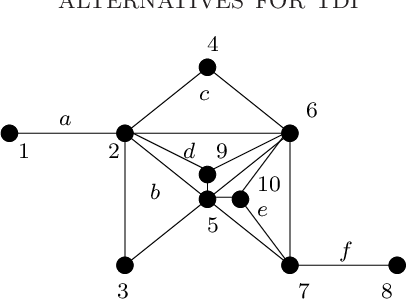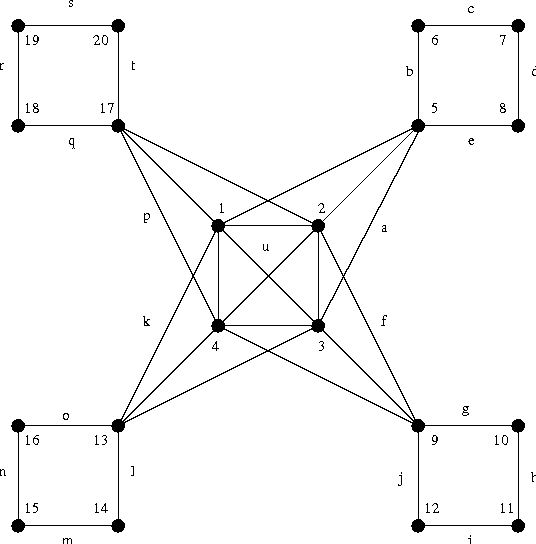You are currently offline. Some features of the site may not work correctly.

# Total dual integrality

Known as: TDI
In mathematical optimization, total dual integrality is a sufficient condition for the integrality of a polyhedron. Thus, the optimization of a… Expand
Wikipedia

## Papers overview

Semantic Scholar uses AI to extract papers important to this topic.
2011
2011
When R is a non-archimedean real closed field we say that a func- tion f 2 R( ¯ X) is finitary at a pointb 2 R n if on some… Expand
Is this relevant?
2008
2008
• Math. Program.
• 2008
• Corpus ID: 6429158.oa
In this paper we provide characterizing properties of totally dual integral (TDI) systems, among others the following: a system… Expand
•Is this relevant?
2007
2007
In this paper we provide new characterizing properties of TDI systems, among others the following: A system of linear… Expand
•Is this relevant?
2001
2001
• R. F.
• 2001
• Corpus ID: 156051039
A linear system Ax < b (A, b rational) is said to be totally dual integral (TDI) if for any integer objective function c such… Expand
Is this relevant?
2000
2000
G=(V, E, A) be a mixed graph. That is, (V, E) is an undirected graph and (V, A) is a directed graph. A matching forest… Expand
Is this relevant?
1995
1995
• Math. Program.
• 1995
• Corpus ID: 12187530
A 0, ±1-matrixA is balanced if, in every submatrix with two nonzero entries per row and column, the sum of the entries is a… Expand
Is this relevant?
1991
1991
• Oper. Res. Lett.
• 1991
• Corpus ID: 122982971.oa
A number of well known results in combinatorial optimization, such as Hoffman's circulation theorem and the matching theorems of… Expand
Is this relevant?
1987
1987
• Math. Program.
• 1987
• Corpus ID: 41326036.oa
We prove that any totally dual integral description of a full-dimensional polyhedron is locally strongly unimodular in every… Expand
Is this relevant?
1981
1981
Abstract We prove that each (rational) polyhedron of full dimension is determined by a unique minimal total dual integral system… Expand
Is this relevant?
1981
1981
Schrijver has shown that any rational polyhedron is the solution set of a unique minimal integer TDI linear system. We… Expand
Is this relevant?